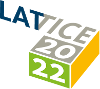#The 39th International Symposium on Lattice Field Theory (Lattice 2022)

Aug 8 – 13, 2022
Hörsaalzentrum Poppelsdorf
Europe/Berlin timezone

## Unpolarized gluon PDF for the proton using the twisted mass formulation

Aug 10, 2022, 4:30 PM
20m
CP1-HSZ/Ground-0.010 - HS2 (CP1-HSZ)

450

### Speaker

Joseph Delmar (Temple University)

### Description

We present results of the x-dependence of the unpolarized gluon PDF for the proton. We use an $N_f = 2+1+1$ ensemble of maximally twisted mass fermions with clover improvement and the Iwasaki improved gluon action. The quark masses are tuned so that the pion mass is $260$ MeV. We use a $32^3 \times 64$ lattice size with a lattice spacing $a=0.093$ fm giving a spatial extent of $3$ fm. We employ the pseudo-distribution approach and obtain the light-cone Ioffe time distribution (ITD) combining data for nucleon momentum boosts up to $1.67$ GeV and Wilson line length, $z$, up to $0.56$ fm. We explore systematic effects such as the dependence on the maximum value of $z$ entering the fits to obtain the ITD. We also study various options to reconstruct the x-dependence of the gluon PDF.

### Primary author

Joseph Delmar (Temple University)

### Co-authors

Constantia Alexandrou Krzysztof Cichy (Faculty of Physics, Adam Mickiewicz University) Martha Constantinou (Temple University) Kyriakos Hadjiyiannakou (University of Cyprus)